Nishant Malhotra Founder of Middle Road OPC Pvt Ltd & The middle Road platform talks about the startup

### Ahoy Ahoy-Ahoy!

Welcome to the Kickass platform enabling social change & impact

# Introduction to Simple Linear Regression

You are here:

### Simple Regression Analysis : An introduction to Linear Regression

This is the first write-up on regression analysis and this topic covers an introduction to simple regression analysis. Simple regression analysis is a bivariate analysis i.e. it has two variables; a statistical technique to fight relationship between variables involving one independent variable and one defendant variable wherein the relationship between the two can be approximated by a straight line. To be more precise the mean response of any value of the regressor variable will be a straight line. This read shares an overview of the simple linear regression model in a nutshell, with an explanation of variables and terminologies within the equation. The note includes an understanding of least square estimation of the coefficients of the regression model

### Least Square Method

The regression analysis is used to estimate causality (cause and effect) between the two variables but points to be noted that a high correlation between two variables does not mean causality. Example shrimps sold in San Francisco might be highly correlated with number of soaps sold in Ghana. This does not does not imply that sale of shrimps is causing the number of soaps sold in Ghana. In this case it could be that another factor is causing increase in sale of shrimps and number of soaps sold or in this hypothetical case used only for illustration purpose purely coincidental. Regression does not imply cause and effect unless the regression has a theoretical consideration outside the sample data analyzed.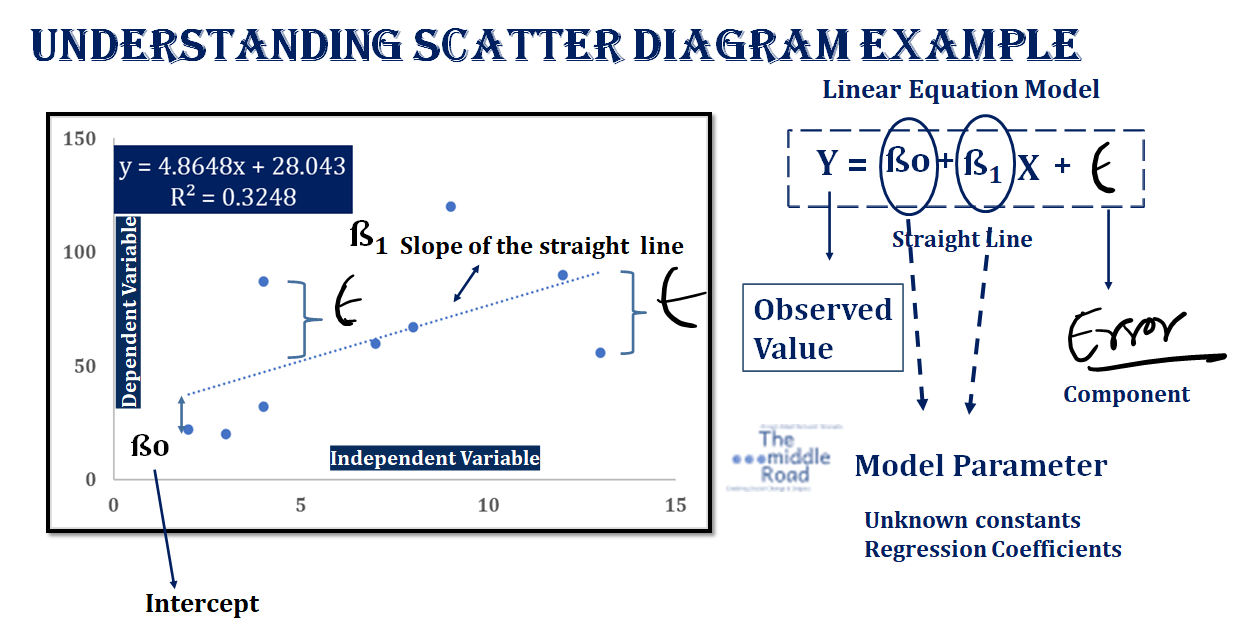Above a graph of bivariate data, wherein the independent variable is on the horizontal axis and the dependent variable is on the vertical axis. In the figure,  simple linear regression model with model parameters and an error component. X is the Independent Variable or Regressor variable or Predictor Variable, Usually approx. of the functional relationship between the two variables. A simple linear equation has only one regressor.

###### Simple Linear Regression Model | The middle Road

The X variable is fixed, the dependent or response variable  is a conditional probability determined by the random component. Since the random component is a normalized curve (with mean of zero and variance σ2), the response variable Y will be a conditional probability normally distributed random variable. This means that the mean response at any value of X is a straight line ßo +  ß1 X.

A detailed video and in-depth module would be following up this read.

### Least Square MethodUnderstand how the coefficients of regression are calculated using the least square model. In simple regression analysis, only one regressor is involved but when there are multiple regressors, we call it multiple regression analysis. Using least square estimation method, regression coefficients can be estimated by minimizing the sum of squares of difference between the observed value and the straight line using the minimum variance concept.  The below derivation is for a simple linear equation with multiple observations. The sample regression model denotes the letter i as subscript used in summation for integers 1,2….n depending on the number of observations. The sample regression model is different from population regression model with the introduction of i as a subscript.

###### Image Simple Linear Equation Diagram | The middle Road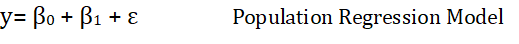Using sample regression model,on the left. i donates the number of observations; i= 1,2,3 …… n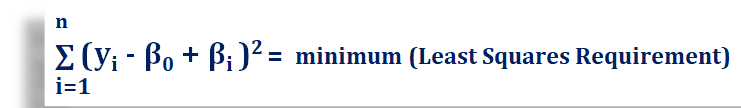Using least square estimation method, regression coefficients can be estimated by minimizing the sum of squares of difference between the observed value and the straight line using the minimum variance concept. The equation on the right will be differentiated with respect to the two coefficients and the first order of the differential equated to zero.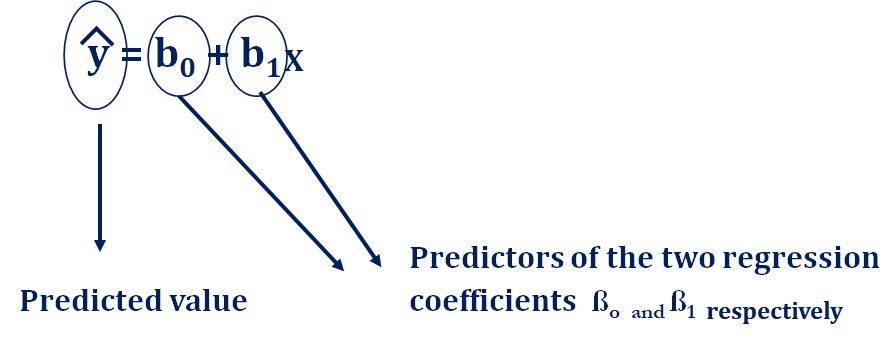The differentiation would be done taking one coefficient parameter at a time. The below example is from the book Introduction to Linear Regression Analysis by Douglas Montgomery,  Elizabeth Peck, G. Geoffrey Vining. However, the complete derivation is solved by The middle Road and written below. Predicted value is also the fitted value.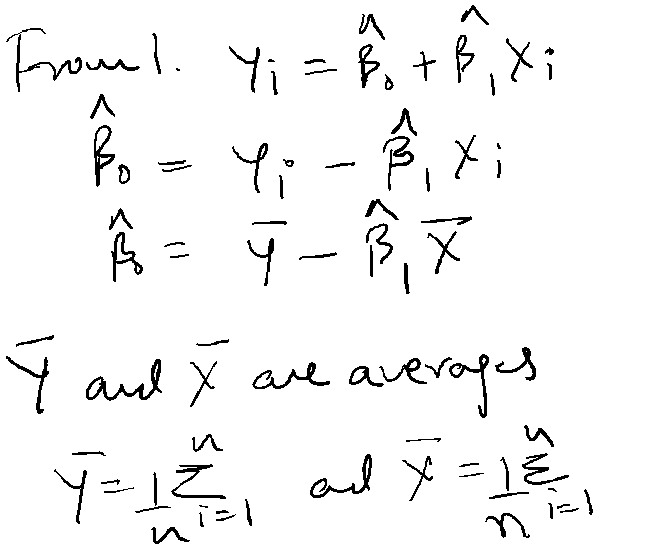Using the above equation 1, we can find the fitted values of both the coefficients of regression. The fitted values of the coefficients of regression are donated with a cap in the equation. The values of Y (Dependent Variable or Response Variable) and X, the Independent/Predictor Variable or Regressor are the averages for the observations of variables in the sample regression model.

Refer to the side equations. Now solving for the other regression coefficient, differentiating and equating the equation to zero.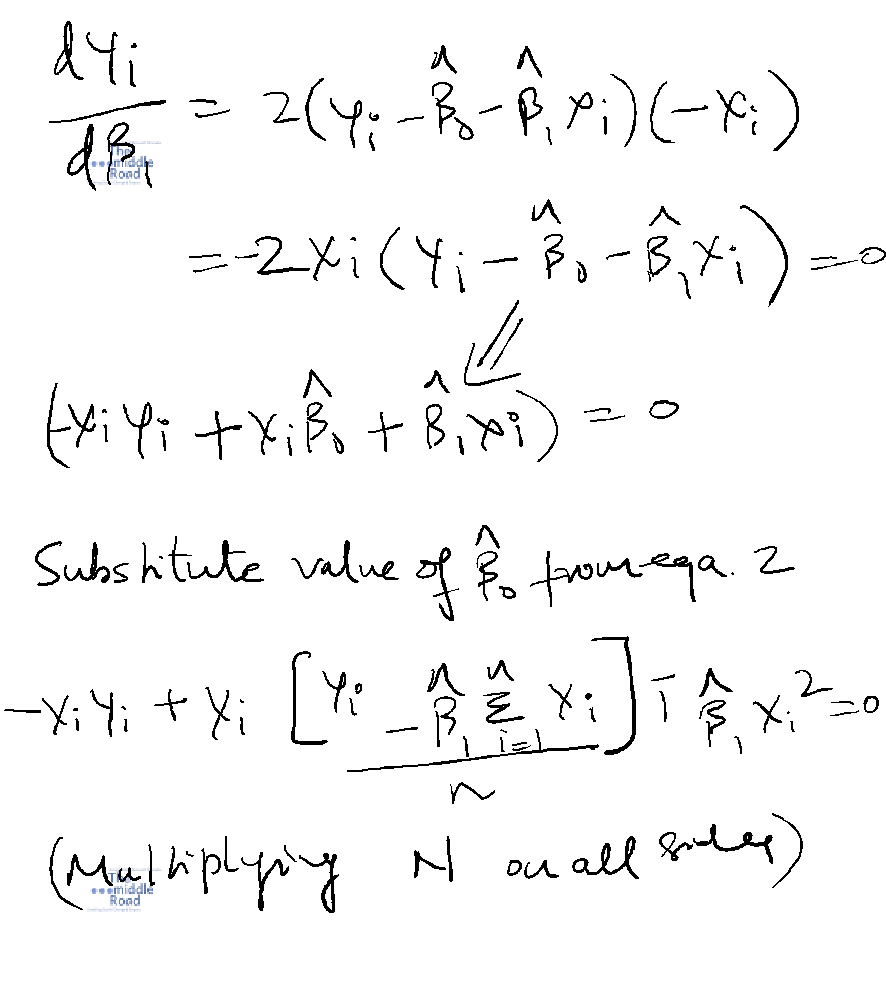The derivation does not include summation sign for the ß1 to making the analysis more simple. The summations are included at the end of the equation.